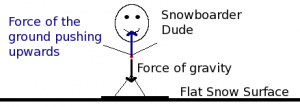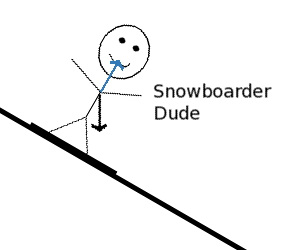# Free Body Diagram

This entry is part of 1 in the series Physics
• Free Body DiagramFree Body Diagram of a Snowboaarder at Rest What we are looking at is a simple free body diagram. Free body diagrams are used by those studying the physics of a situation to understand and visualize the forces at play in the situation under study.

In the situation I depict above, a snowboarder is on a level surface, that is the surface is perpendicular to the direction of the force of gravity. The black arrow represents the force of gravity and the blue arrow is the force of the snow-covered earth pushing back against the snowboarder! The last part seems a bit counter-intuitive and you need to think about it.

In this case, the snowy surface of the earth pushes against the skier perpendicular to the surface — usually referred to as force normal. Without that force normal our snowboarder is in free fall!

There are a few assumptions at play here and all of them suit our needs quite well. We assume a flat earth model which is reasonable because we are small and very close to the earth’s surface, this makes calculations much easier.

However, I suppose your next question is what does the situation look like when our stick snowboarder is going down a slope? Good question, yes the diagram differs. Let us assume our snowboarding friend (and yes, they are our friends) is zooming down a run with a grade of about 58% (30°), that would appear as:Freebody Diagram Showing Forces on a 30° Run Notice now how the force of gravity (black arrow) and the force normal (blue arrow) do not line up anymore? They no longer entirely cancel each other out. We now break the gravitational force into two constituent parts, one component is parallel to the trail and the other is perpendicular to the trail.

The normal force balances the perpendicular force component, but the parallel component has NO opposing force (we ignore wind resistance and frictional forces, at least for now) and where there is an unopposed force there is acceleration!

How much acceleration? Well, the force = mass x acceleration. Gravitational acceleration is 9.8 meters/sec2 x mass (in kilograms). However, in the last case we also need to multiply that by the sine of 30° (which is .5). So, let us say for myself, I weigh 220 lbs (100 kg, nice round number) this would work out to a force of 9.8*(100/2)= 980/2 = 490 Newtons.

So, a force of 490 newtons would be driving me down this ski hill. We would then take that force apply it to my mass to determine the acceleration down the hill and using that result we could determine my velocity at various points in time.

I will tell you one thing, 30° does not seem like much of a hill, but skiing last weekend MyTracks reports my minimum grade as being about 34% and that translates into a 20° angle of descent and that would be pushing the steepest runs I have been on.

#### Be the first to comment

This site uses Akismet to reduce spam. Learn how your comment data is processed.